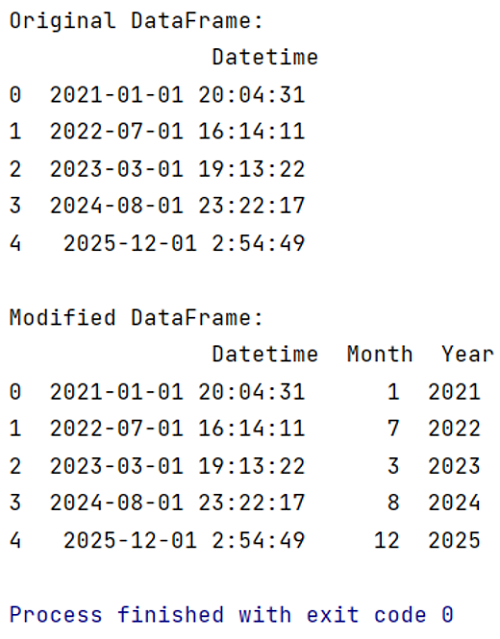# How to extract month and year separately from datetime in pandas?

Learn how to extract month and year separately from datetime in pandas?
Submitted by Pranit Sharma, on May 10, 2022

The datetime is a library in Python which is a collection of date and time. Inside Datetime, we can access date and time in any format, but usually date is present in the format of "yy-mm-dd" and time is present in the format of "HH:MM:SS’".

Here,

• yy means year
• mm means month
• dd means day
• HH means hours
• MM means minutes
• SS means seconds

While accessing the date and time from datetime, we always get date and time together, here we are going to learn how to extract month and year separately DateTime?

To work with pandas, we need to import pandas package first, below is the syntax:

```import pandas as pd
```

Let us understand with the help of an example:

```# Importing datetime package
import pandas as pd

# Importing datetime package
import datetime

# Creating a dictionaries of datetime
d = {'Datetime':['2021-01-01 20:04:31',
'2022-07-01 16:14:11',
'2023-03-01 19:13:22',
'2024-08-01 23:22:17',
'2025-12-01 2:54:49']}

# Creating a DataFrame
df = pd.DataFrame(d)

# Display original DataFrame
print("Original DataFrame:\n",df,"\n")

# Extracting month and year from Datetime
# Adding a new column named Month & year
# having months and years only
df['Month'] = pd.to_datetime(df['Datetime']).dt.month

df['Year'] = pd.to_datetime(df['Datetime']).dt.year

# Display modified DataFrame
print("Modified DataFrame:\n",df)
```

Output:Preparation